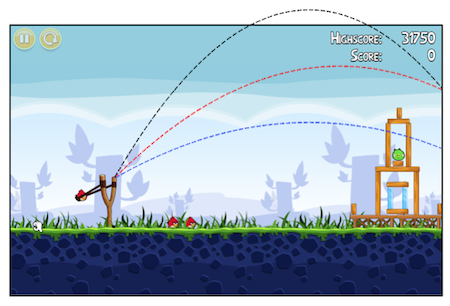# Projectile and Kinetic energy combinedA projectile thrown from horizontal has half the kinetic energy when it is at $h = \dfrac{3H_\text{max}}{4}$ than when it is at $h = \dfrac{H_\text{max}}{4}$.

If the angle of projection can be written as $\theta = \tan^{-1}x$, find the value of $x$.

×

Problem Loading...

Note Loading...

Set Loading...